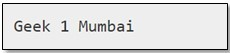# Convert JSON data Into a Custom Python Object

• Last Updated : 16 Dec, 2021

Let us see how to convert JSON data into a custom object in Python. Converting JSON data into a custom python object is also known as decoding or deserializing JSON data. To decode JSON data we can make use of the json.loads(), json.load() method and the object_hook parameter. The object_hook parameter is used so that, when we execute json.loads(), the return value of object_hook will be used instead of the default dict value.We can also implement custom decoders using this.
Example 1 :

## Python3

 `# importing the module``import` `json``from` `collections ``import` `namedtuple` `# creating the data``data ``=` `'{"name" : "Geek", "id" : 1, "location" : "Mumbai"}'` `# making the object``x ``=` `json.loads(data, object_hook ``=``               ``lambda` `d : namedtuple(``'X'``, d.keys())``               ``(``*``d.values()))` `# accessing the JSON data as an object``print``(x.name, x.``id``, x.location)`

Output :As we can see in the above example, the namedtuple is a class, under the collections module. It contains keys that are mapped to some values. In this case, we can access the elements using keys and indexes. We can also create a custom decoder function, in which we can convert dict into a custom Python type and pass the value to the object_hook parameter which is illustrated in the next example.
Example 2 :

## Python3

 `# importing the module``import` `json``from` `collections ``import` `namedtuple` `# customDecoder function``def` `customDecoder(geekDict):``    ``return` `namedtuple(``'X'``, geekDict.keys())(``*``geekDict.values())` `# creating the data``geekJsonData ``=` `'{"name" : "GeekCustomDecoder", "id" : 2, "location" : "Pune"}'` `# creating the object``x ``=` `json.loads(geekJsonData, object_hook ``=` `customDecoder)` `# accessing the JSON data as an object``print``(x.name, x.``id``, x.location)`

Output :We can also use SimpleNamespace class from the types module as the container for JSON objects. Advantages of a SimpleNamespace solution over a namedtuple solution: –

1. It is faster because it does not create a class for each object.
2. It is shorter and simpler.

Example 3 :

## Python3

 `# importing the module``import` `json``try``:``    ``from` `types ``import` `SimpleNamespace as Namespace``except` `ImportError:``    ``from` `argparse ``import` `Namespace` `# creating the data``data ``=` `'{"name" : "GeekNamespace", "id" : 3, "location" : "Bangalore"}'` `# creating the object``x ``=` `json.loads(data, object_hook ``=` `lambda` `d : Namespace(``*``*``d))` `# accessing the JSON data as an object``print``(x.name, x.``id``, x.location)`

Output :My Personal Notes arrow_drop_up